﻿ The Solution Of The Problem. Correct Solution. Flowchart. 3D Illustration Stock Illustration - Illustration of illustration, science: 84869199

The solution of the problem. Correct solution. Flowchart. 3D illustration. Science, solve.

The solution of the problem. Correct solution. Flowchart.

More similar stock illustrationsProblem and solutionSolution of problem in caseOffice Cooperative Teamwork. People Build Puzzles. Problem Solution Business Concept Vector Illustration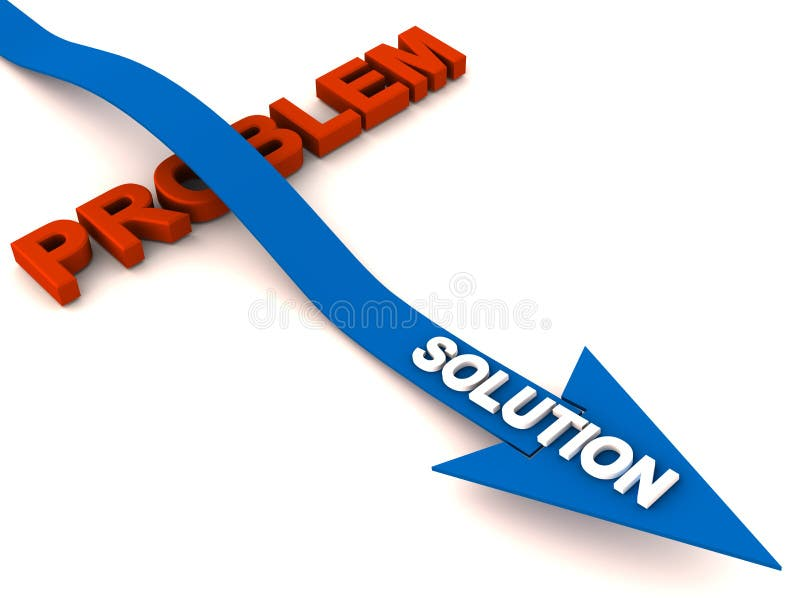Overcome problem with solutionCouple having idea. Husband and wife have solution, adult male and female solved problem or ideas lamp cartoon vector3d problem and solutionAmbiguity, person, problem, solution, question concept. Hand drawn isolated vector.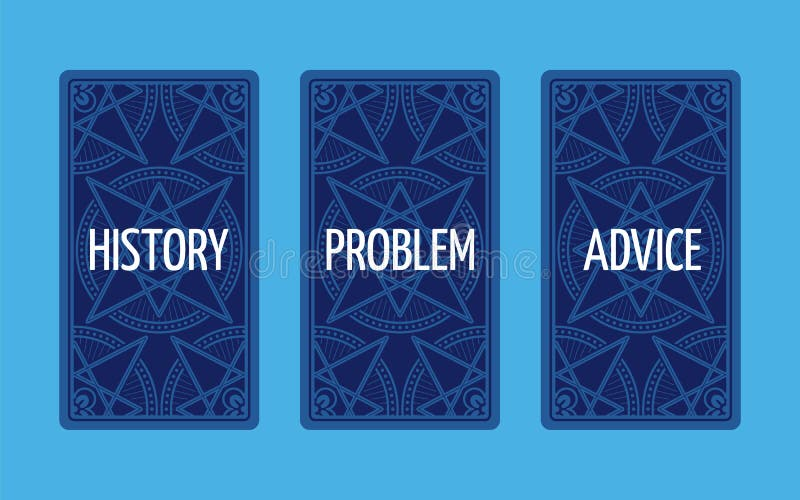Three card tarot spread. Problem solution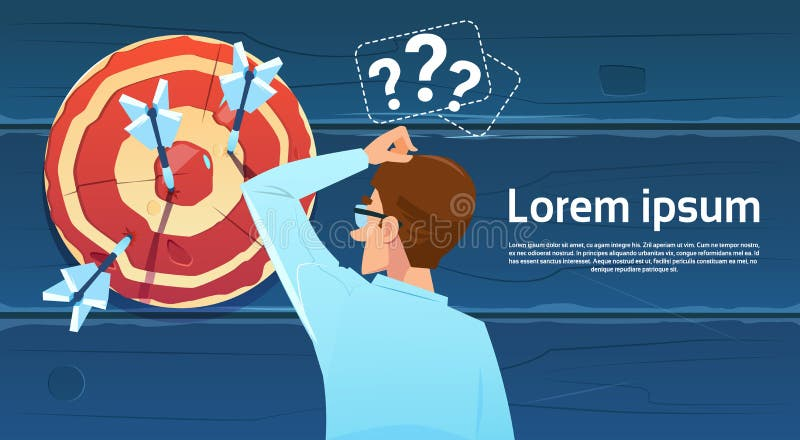Confused Business Man Standing Back Looking At Target Aim Question Mark Think Problem Solution
More stock photos from Alexander Kaverin's portfolio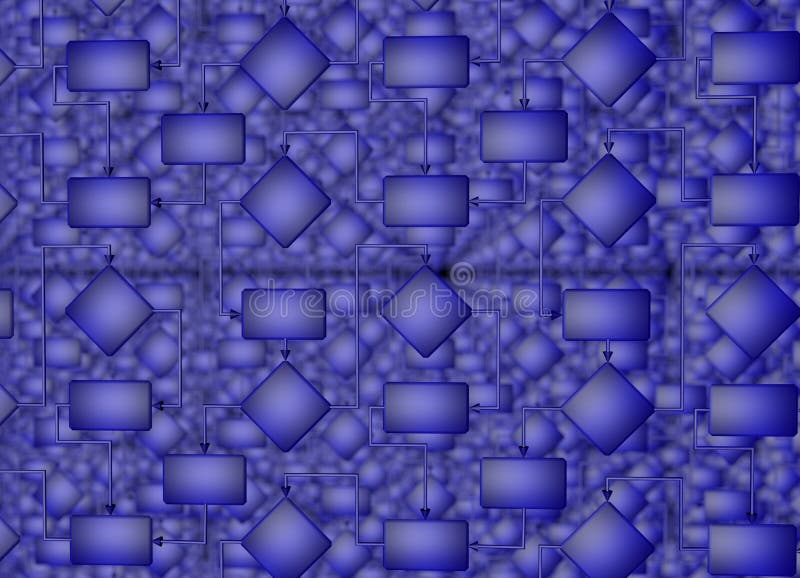The solution of the problem. Correct solution. Flowchart. 3D illustration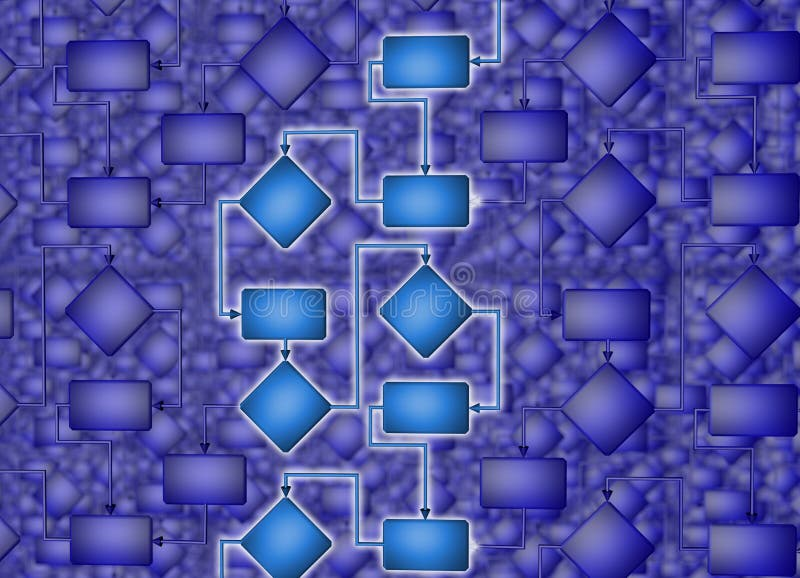The solution of the problem. Correct solution. Flowchart. 3D illustration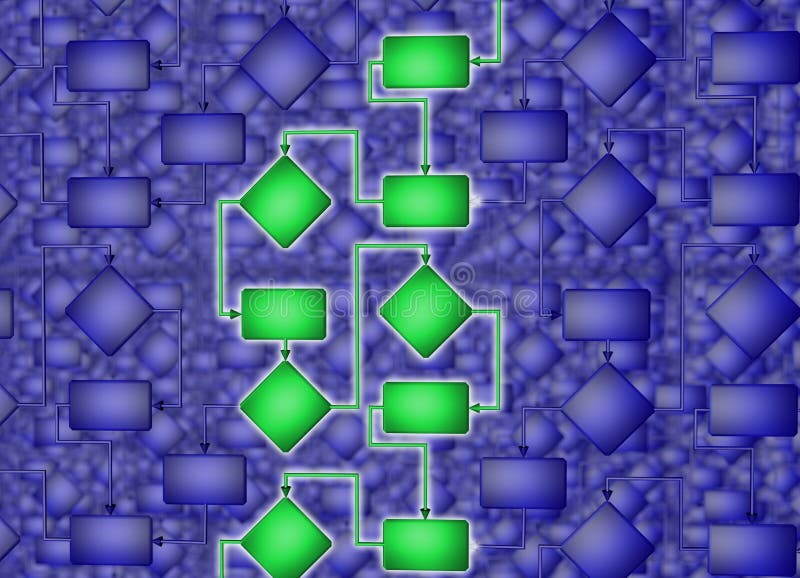The solution of the problem. Correct solution. Flowchart. 3D illustration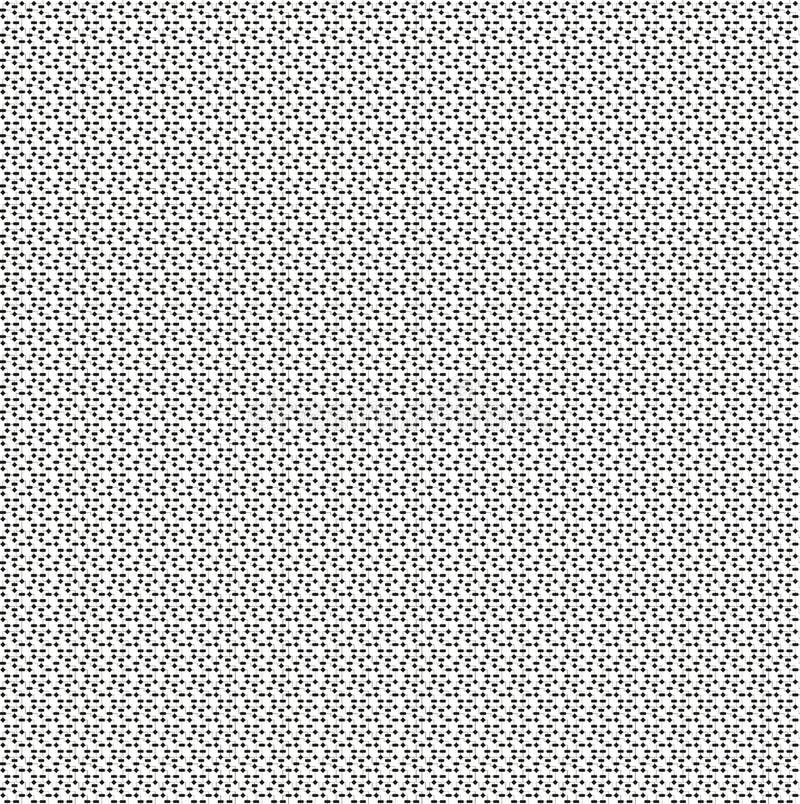Flowchart. The solution of the problem. Correct solution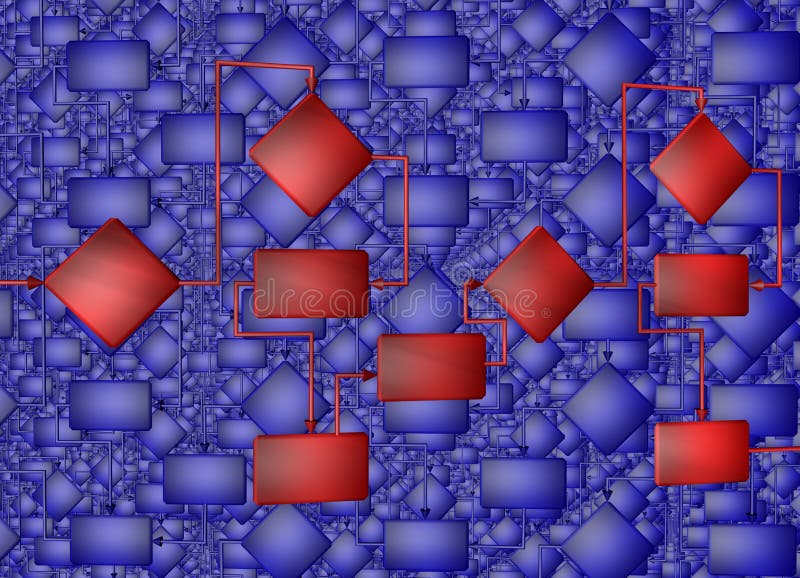The solution of the problem. Correct solution. Flowchart. 3D illustration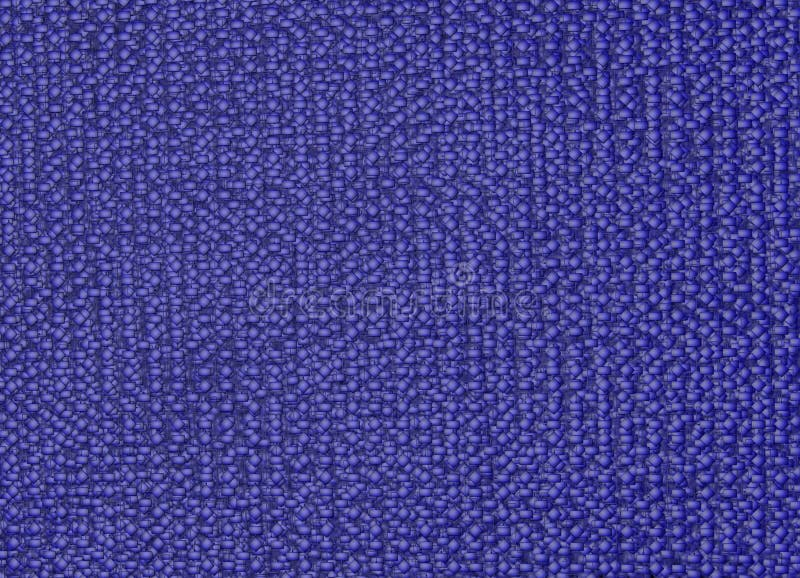The solution of the problem. Correct solution. Flowchart. 3D illustration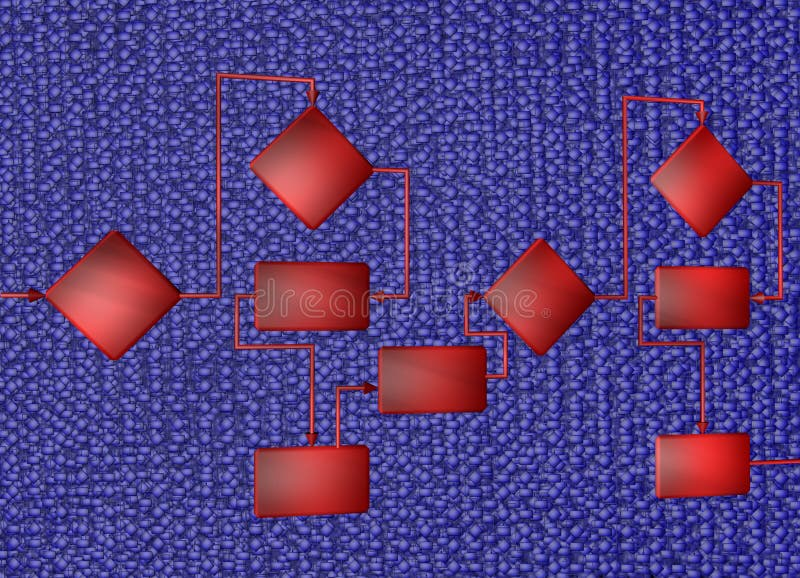The solution of the problem. Correct solution. Flowchart. 3D illustration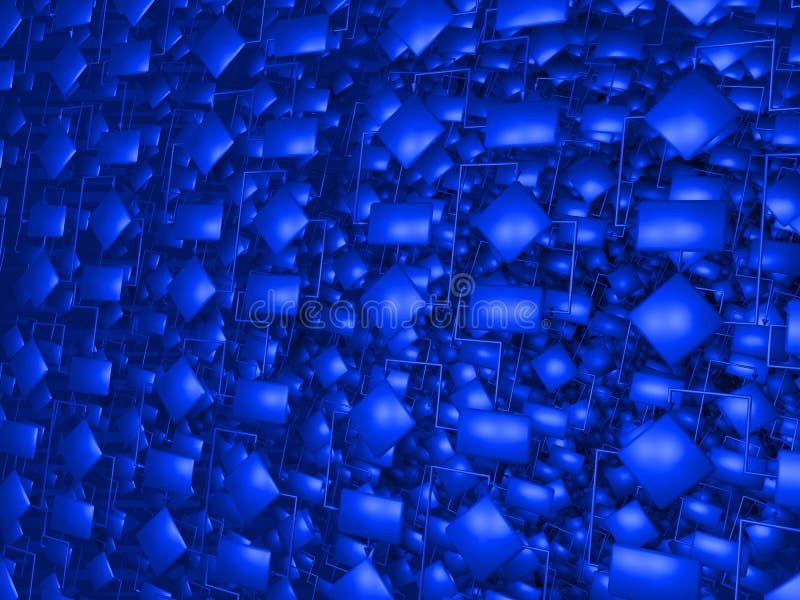The solution of the problem. Correct solution. Flowchart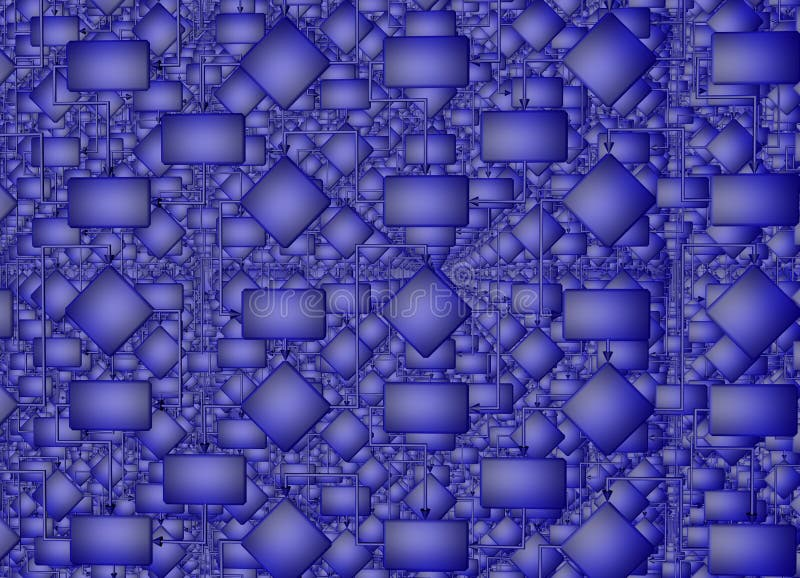The solution of the problem. Correct solution. Flowchart. 3D illustration